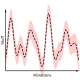# Two Fibers, Five Ways

I occasionally go down rabbit holes of numerical integration. These trips led me to Gauss-Radau integration, all the element integration options available in OpenSees, and, recently, Chebyshev integration.

The latest rabbit hole, described in this post, deals with different ways to integrate section moment-curvature response using only two fibers.

Consider a rectangular cross-section with EPP stress-strain response and no axial load. We seek the moment-curvature response about the local z axis.

Although not shown here, the exact moment-curvature relationship is easily obtained in closed-form.

To compute the moment-curvature response using only two fibers, I can think of five integration rules that chop this section into two pieces with prescribed sample point locations and areas.

• Midpoint – Two fibers of equal area and sample points at the geometric center of each area. This is the approach implemented for patches in OpenSees.
• Gauss – Map two-point Gauss integration through the section depth. The fibers have equal area but the sample points move away from the geometric center of each fiber.
• Lobatto – Map two-point Gauss-Lobatto integration through the section depth. This approach gives fibers of equal area but the sample points are at the edges of the section.
• Radau – Map two-point Gauss-Radau integration through the section depth. This approach gives unequal fiber areas and asymmetric sample points.
• Newton-Cotes – Map an open two-point Newton-Cotes rule through the section depth. This approach gives equal fiber areas and the sample points are located at 1/3 points through the section depth.

The normalized moment-curvature response computed with each two fiber integration rule is shown below.

Note that identifying information as to which plot corresponds to which integration rule has been removed. In the comments section below, match the integration rules with the computed results, e.g., “1-Gauss, 2-Midpoint, …”. There are 120 possible matching sequences, so think it through! And feel free to share your logic with your response.

I will explain the correct answers in a follow up post. Responses are due by January 31, 2022.

If you want to try this out for yourself in OpenSees, use the `fiber` command to manually define the area and sample point location of individual fibers. To avoid numerical issues with EPP, I used a very small strain-hardening ratio in the material stress-strain response. I also did not restrain the axial DOF for the moment-curvature analyses.

RESULTS

## 6 thoughts on “Two Fibers, Five Ways”

1.gopal adhikari says:

Hi,

My two-cents are

1- Lobatto; 2-Gauss; 3-Radau; 4-Newton Cotes; 5-Midpoint

Liked by 1 person

2.Mark Denavit says:

I also think
1. Lobatto
2. Gauss
4. Newton-Cotes
5. Midpoint

Liked by 1 person

3.Luigi says:

By looking only at the final value of convergence and comparing the lever arm with the exact one:
1: Lobatto
2. Gauss
5. Midpoint

And looking at the stiffness, Newton-Cotes has a smaller distance between the two centers so it must have a smaller stiffness and be number 4. And Radau number 3.
However looking at the stiffness, I would say that Gauss should have a slightly smaller stiffness than the exact stiffness. So my guess is that (the correct) Gauss is not there.

Liked by 1 person

4.Hakki Deniz Gul says:

Greetings,

I created a simple python script to perform the analysis. The result is as given in the comments of Gopal and Mark.

You can check the python script from the link: https://github.com/hakki-deniz/Moment-Curvature

Note: I obtained the exact solution by using patch command (10×10 fibers).

A question : Which software do you use to draw these simple and neat figures?

Thank you !

Liked by 1 person

1.Michael H. Scott says:

Thank you! I use xfig.

Like

This site uses Akismet to reduce spam. Learn how your comment data is processed.